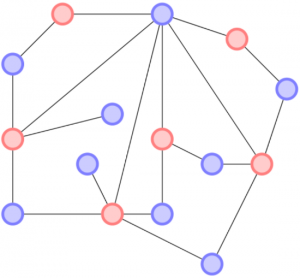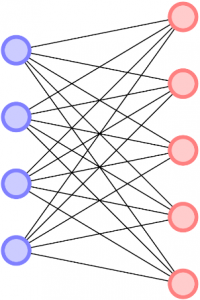By Patrick Adams, La Trobe UniversityA graph is a mathematical tool used to describe the relationships between objects. Graphs are most commonly shown as a drawing, where objects are displayed as vertices, or points on a plane, and the relationships are displayed as edges, lines connecting the vertices.

There is a lot of terminology associated to graph theory, most importantly related to my research project are the words degree and degree sequence. The degree of a vertex is how many edges are connected to it. The degree sequence is the sequence of numbers of all the degrees of all the vertices in a graph.

In figure 1, the degree of the topmost blue vertex is 6. If we took all the degrees in this graph, the degree sequence would be {6, 26, 12| 5, 42, 3, 22}. The powers aren’t really powers in this sequence, they just note how many of that degree vertex appear. So 26 isn’t a 64 degree vertex, it means there are six, 2 degree vertices. The | also signifies the separations of parts. Hopefully you have noticed there two colours, or parts of the graph. You may have also noticed that there are no blue vertices connected to blue vertices, and no red vertices connected to red vertices. This makes the graph bipartite.In figure 2, we see another bipartite graph. But alas, this graph is different again! Firstly we see that the degree sequence is {45|54}, it only has two types of degrees. If you look carefully, you’ll see that all vertices of the same colour have the same degree! This is a special type of graph that is the focus of my report, BB graphs. BB stands for Bi-regular, only two elements in the degree sequence, and bipartite.

We notice that there is a lot of edge crossing in this drawing. Interestingly, this degree sequence will never have a graph without edge crossing. To find out why, and how to tell if other BB graphs can be drawn without edge crossing, you’ll have to read my research project!

Patrick Adams was one of the recipients of a 2017/18 AMSI Vacation Research Scholarship.